﻿ 基于数值仿真的螺旋桨盘面比对船舶自航因子影响分析
 舰船科学技术2022, Vol. 44Issue (10): 33-36    DOI: 10.3404/j.issn.1672-7649.2022.10.007PDF

, 楼丹平4, 陈炯5, 宋磊1,2,3, 童骏6
1. 华中科技大学 船舶与海洋工程学院，湖北 武汉 430074;
2. 船舶数据技术与支撑软件湖北省工程研究中心，湖北 武汉 430074;
3. 高新船舶与深海开发装备协同创新中心，上海 200240;
4. 沪东中华造船(集团)有限公司开发研究所，上海 200129;
5. 九江职业技术学院 船舶与海洋工程技术研究所，江西 九江 332000;
6. 武汉第二船舶设计研究所 湖北 武汉 430064

Numerical simulation of model scale self-propulsion with different area ratio of the propeller
LIU Zeng1,2,3,4, LOU Dan-ping4, CHEN Jiong5, SONG Lei1,2,3, TONG Jun6
1. School of Naval and Ocean Engineering, Huazhong University of Science and Technology, Wuhan 430074, China;
2. Hubei Engineering Research Center of Ship Data Technology and Support Software, Wuhan 430074, China;
3. Collaborative Innovation Center for High-tech Ships and Deep-sea Development Equipment, Shanghai 200240, China;
4. Development Research Institute in Hudong Zhonghua Shipbuilding (Group) Co. Ltd., Shanghai 200240, China;
5. Institute of Ship and Marine Engineering Technology in Jiujiang Polytechnic, Jiujiang 332000, China;
6. Wuhan Second Ship Design and Research Institute, Wuhan 430064, China
Abstract: Taking KCS ship and KP505 propeller as objects, complete the three-dimensional model establishment, mesh division, boundary condition setting and flow field solution according to a certain scale ratio. The numerical calculation results are compared with the existing test results to verify the accuracy of the numerical calculations. According to the verified numerical calculation scheme, the self-propelled calculation of the KCS ship with different disc ratio propellers was completed. The results showed that the hull resistance and thrust deduction did not change significantly under the action of different disc ratio propellers, but the total propulsion efficiency increased with The increase of the paddle-to-disk surface ratio shows a decreasing trend.
Key words: numerical simulation     self-propulsion factor     propeller disc ratio
0 引　言

1 数值计算原理

 $\nabla \cdot \mathit{u}=0 \text{，}$ (1)
 $\frac{\partial {u}_{i}}{\partial t}+\frac{\partial \left({u}_{i}{u}_{j}\right)}{\partial {x}_{j}}=-\frac{1}{\rho }\frac{\partial p}{\partial {x}_{i}}+\nu \frac{\partial }{\partial {x}_{j}}\left(\frac{\partial {u}_{i}}{\partial {x}_{j}}+\frac{\partial {u}_{j}}{\partial {x}_{i}}\right) \text{。}$ (2)

 $22{u}_{i}=\overline{{u}_{i}}+{u}_{i}\mathrm{\text{′}} \text{，}$ (3)

 $\frac{\partial {u}_{i}}{\partial t}+\frac{\partial \left({u}_{i}{u}_{j}\right)}{\partial {x}_{j}}=-\frac{1}{\rho }\frac{\partial \rho }{\partial {x}_{i}}+\nu \frac{\partial }{\partial {x}_{j}}\left(\frac{\partial {u}_{i}}{\partial {x}_{j}}+\frac{\partial {u}_{j}}{\partial {x}_{i}}\right)+\frac{1}{\rho }\frac{\partial }{\partial {x}_{j}}(-\overline{{u}_{i}\mathrm{\text{′}}{u}_{j}\mathrm{\text{′}}}) \text{。}$ (4)
2 网格及边界条件设置表 1 KCS集装箱船主要参数 Tab.1 Main parameters of KCS container ship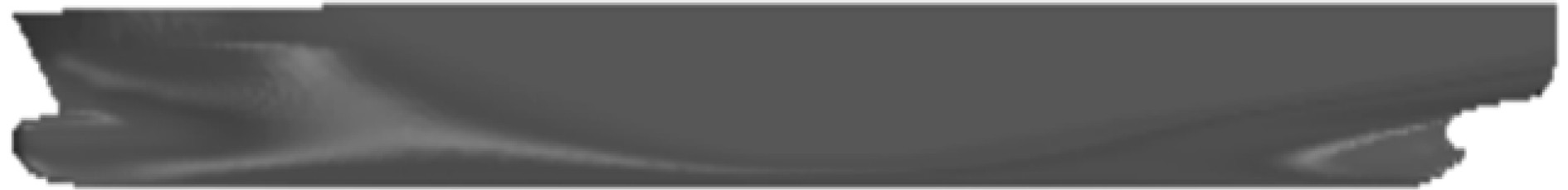图 1 KCS集装箱船模型 Fig. 1 KCS container ship model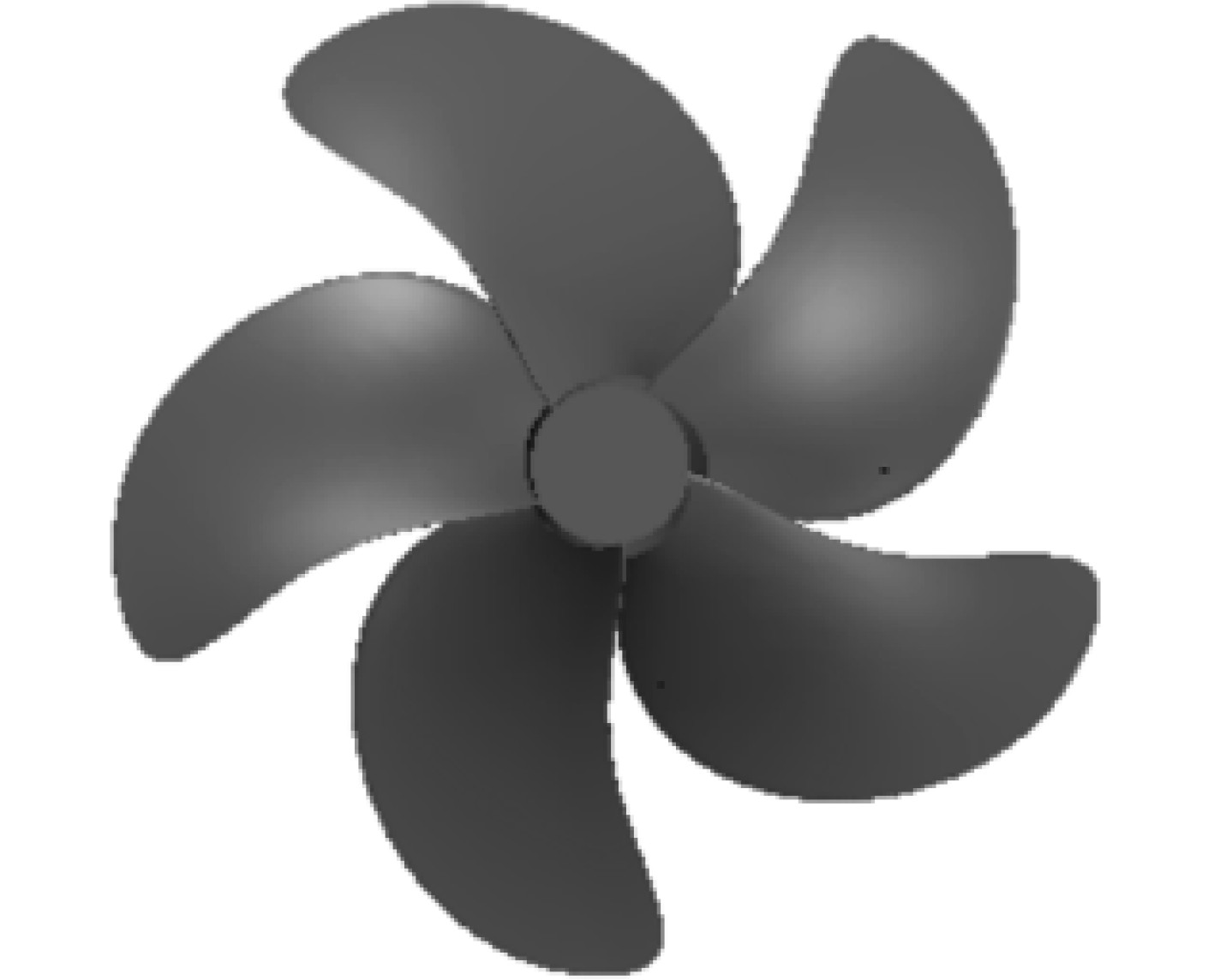图 2 KP505桨模型 Fig. 2 KP505 propeller model
2.1 计算域网格划分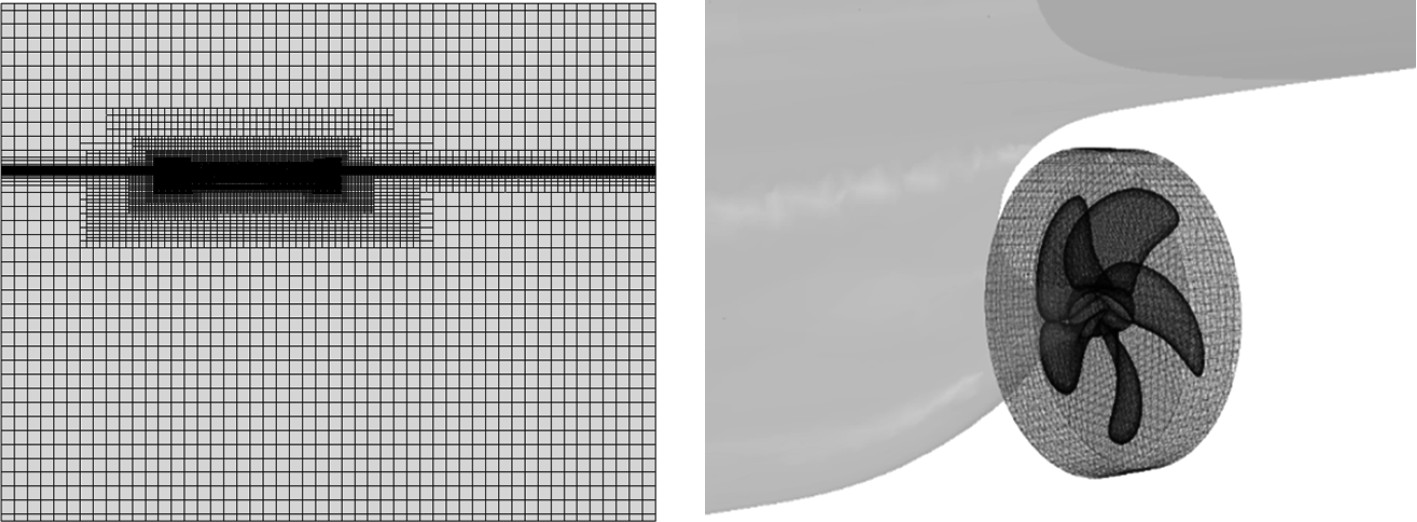图 3 自航计算旋转域网格 Fig. 3 Self-propelled computing rotation domain grid
2.2 边界条件设置

3 结果分析 3.1 KP505桨计算结果表 2 不同转速船桨受力结果 Tab.2 Force results of propellers at different speeds表 3 自航模拟与实验结果对比1 Tab.3 Comparison of self-propelled simulation and experimental results 1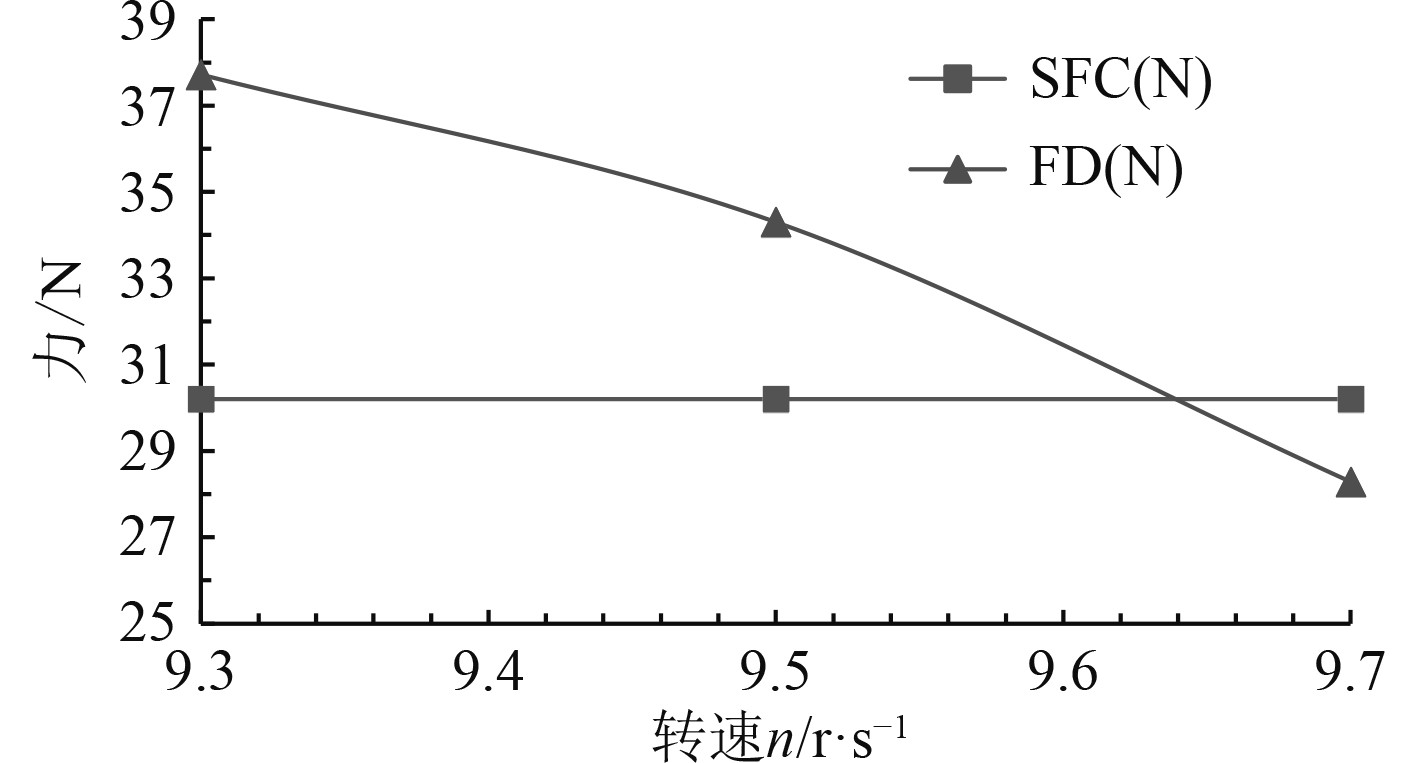图 4 自航曲线 Fig. 4 Self-propelled curve表 4 自航模拟与实验结果对比2 Tab.4 Comparison of self-propelled simulation and experimental results 2

3.2 不同盘面比桨计算结果表 5 装配不同桨自航模拟结果1 Tab.5 Self-propelled simulation results with different propellers 1表 6 装配不同桨自航模拟结果2 Tab.6 Self-propulsion simulation results with different propellers 2

3种盘面比螺旋桨自航计算船尾动压力云图如图5所示，x/L = 0.99处盘面轴向速度分布对比如图6所示。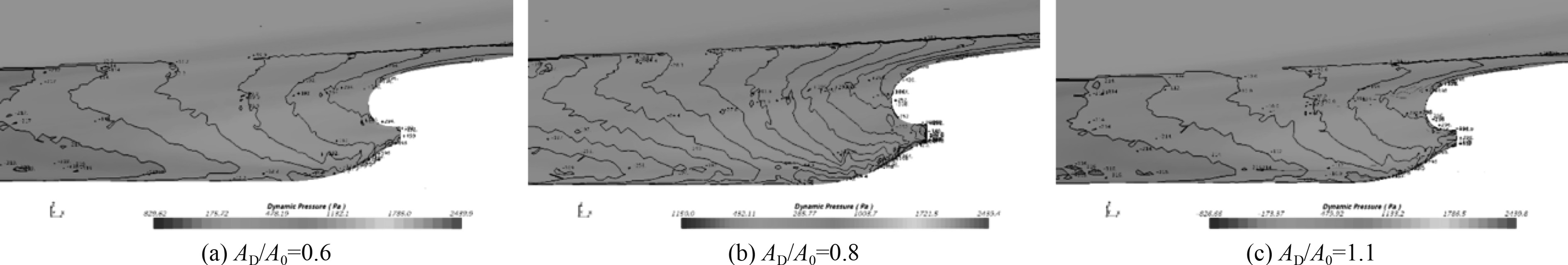图 5 不同盘面比下船尾表面动压力云图 Fig. 5 The dynamic pressure on the stern surface under different disk surface ratios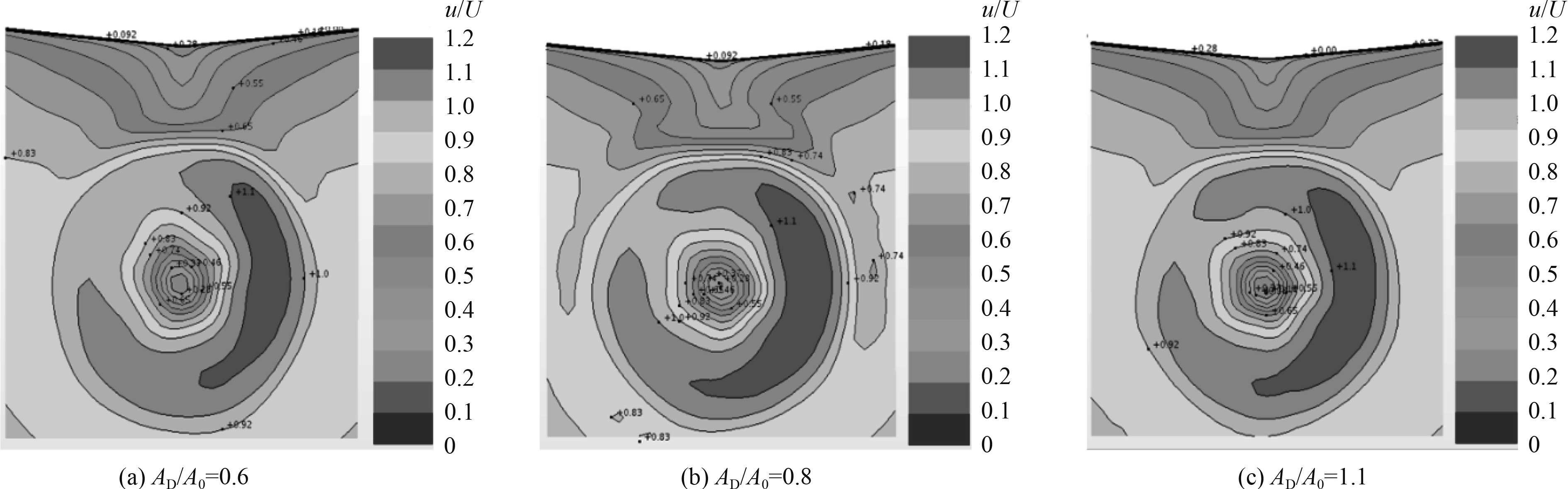图 6 不同盘面比轴向速度分布对比 Fig. 6 Comparison of axial velocity distribution of different disk surface ratios

4 结　语

1）以本文方案进行船舶阻力和自航数值仿真，自航船体阻力误差在1%以内，自航因子误差在5%以内，可知结果可信度较高，能满足工程应用需求。

2）不同盘面比下船体阻力变化不显著，推力减额变化不显著，但总推进效率随着桨盘面比增大呈减小趋势，主要原因是随着盘面比增大螺旋桨自身效率降低，同时伴流减小造成船身效率减小。

  何惠明. 船艇波浪中自航试验研究[J]. 上海船舶运输科学研究所学报, 2005(1): 3-10. DOI:10.3969/j.issn.1674-5949.2005.01.001  CARRICA P M, CASTRO A M, STERN F. Self-propulsion computations using a speed controller and a discretized propeller with dynamic overset grids[J]. Journal of Marine Science and Technology, 2010, 15(4): 316-330. DOI:10.1007/s00773-010-0098-6  CASTRO A M, CARRICA P M, STERN F. Full scale self-propulsion computations using discretized propeller for the KRISO container ship KCS[J]. Computers & Fluids, 2011, 51(1): 35-47.  郭春雨, 赵庆新, 赵大刚. 基于CFD仿真模拟的船模自航试验数据处理[J]. 船海工程, 2013, (3): 17−20.  沈兴荣. 船模自航试验数值模拟研究[C]// 第十一届全国水动力学学术会议暨第二十四届全国水动力学研讨会并周培源诞辰110周年纪念大会, 2012: 1082−1090.  胡俊明, 李铁骊, 林焰, 等. 基于RANS法螺旋桨毂帽鳍水动力性能数值研究[J]. 船海工程, 2015(1): 52-55. DOI:10.3963/j.issn.1671-7953.2015.01.013  TAHARA Y, WILSON R V, CARRICA P M, et al. RANS simulation of a container ship using a single-phase level-set method with overset grids and the prognosis for extension to a self-propulsion simulator[J]. Journal of Marine Science and Technology, 2006, 11(4): 209-228. DOI:10.1007/s00773-006-0231-8  张志荣, 李百齐, 赵峰. 船舶粘性流动计算中湍流模式应用的比较[J]. 水动力学研究与进展(A辑), 2004(5): 637-642.  RAVEN H C, VAN DER PLOEG A, STARKE A R, et al. Towards a CFD-based prediction of ship performance-progress in predicting full-scale resistance and scale effects[J]. International Journal of Maritime Engineering, 2008, 150: A4.  黄少锋, 张志荣, 赵峰, 等. 带自由面肥大船粘性绕流场的数值模拟[J]. 船舶力学, 2008(1): 46-53. DOI:10.3969/j.issn.1007-7294.2008.01.007  BHUSHAN S, XING T, CARRICA P, et al. Model-and full-scale URANS simulations of Athena resistance, powering, seakeeping, and 5415 maneuvering[J]. Journal of Ship Research, 2009, 53(4): 179-198. DOI:10.5957/jsr.2009.53.4.179